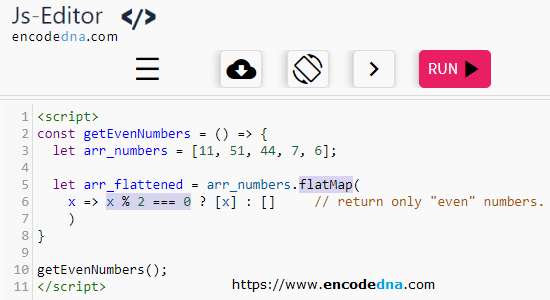﻿ Find Even numbers in Array using flatMap() in JavaScript

# Find Even numbers in Array using flatMap() in JavaScript

There are few simple methods in JavaScript to filter even or odd numbers in an Array. Like you can loop through each number in the array and check whether the number odd or even or in the array and check whether the number odd or even or you can use the built-in filter() method to do the same. However, there’s another interesting built-in method in JavaScript called flatMap() that you can use to quickly filter or find even (or odd) numbers in an array.What is flatMap() method in JavaScript? I have already explained about it in detail in my previous article. Go through it please.

Now, let’s see how we can extract or filter out only even numbers from an array.

## The Script

```<script>
const getEvenNumbers = () => {
let arr_numbers = [4,5,7,8,14,45,76];
let arr_flattened = arr_numbers.flatMap(
x => x % 2 === 0 ? [x] : []		// filter and return only "even" numbers.
)

console.log(arr_flattened);       // Output: 4,8,14,76
}

getEvenNumbers();
</script>```

// Use this code to get odd numbers.

x => x % 2 === 1 ? [x] : []